Courses

# Solutions of Refraction of Light (Page No- 246) - Physics By Lakhmir Singh, Class 10 Class 10 Notes | EduRev

## Class 10 : Solutions of Refraction of Light (Page No- 246) - Physics By Lakhmir Singh, Class 10 Class 10 Notes | EduRev

The document Solutions of Refraction of Light (Page No- 246) - Physics By Lakhmir Singh, Class 10 Class 10 Notes | EduRev is a part of the Class 10 Course Lakhmir Singh & Manjit Kaur Solutions: Class 10 Science.
All you need of Class 10 at this link: Class 10

Page No:246

Question 1: Write the formula for a lens connecting image distance (υ),object distance (u)and the focal length (ƒ).How does the lens formula differ from the mirror formula ?

Solution : Formula for a lens connecting image distance (v). object distance (u) and the focal length (f) is: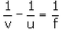This is the lens formula.

The lens formula has a minus sign (-) between 1/v and 1/u whereas the mirror formula has a plus sign (+) between 1/v and 1/u.

Mirror formula: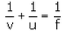Question 2: Write down the magnification formula for a lens in terms of object distance and image distance. How does this magnification formula for a lens differ from the corresponding formula for a mirror ?

Solution : Magnification (m) fomula for a lens is: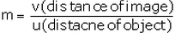Magnification formula for a mirror has a minus sign (-) but the magnification formula for a lens has no minus sign.

Magnification formula for a mirror is: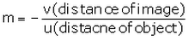Question 3: What is the nature of the image formed by a convex lens if the magnification produced by the lens is +3 ?

Solution : The image will be virtual and erect, since the magnification has positive value.

Question 4: What is the nature of the image formed by a convex lens if the magnification produced by the lens is, – 0.5 ?

Solution : The image will be real and inverted, since the magnification has negative value.

Question 5: What is the position of image when an object is placed at a distance of 10 cm from a convex lens of focal length 10 cm ?

Solution : u = -10 cm, f = 10 cm

we have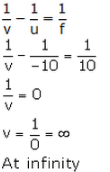Question 6: Describe the nature of image formed when an object is placed at a distance of 30 cm from a convex lens of focal length 15 cm.

Solution : Since the object is placed at a distance greater than the focal length of the convex lens, so the image formed is real and inverted.

Question 7: At what distance from a converging lens of focal length 12 cm must an object be placed in order that an image of magnification 1 will be produced ?

Solution :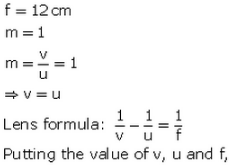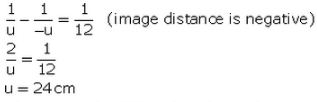The object should be placed at a distance of 24 cm to from the lens (on the left side).

Question 8: State and explain the New Cartesian Sign Convention for spherical lenses.

Solution : New Cartesian Sign Convention for spherical lenses:
(i) All the distances are measured from the optical centre of the lens.
(ii) The distances measured in the same direction as that of incident light are taken as positive.
(iii) The distances measured against the direction of incident light are taken as negative.
(iv) The distances measured upward and perpendicular to the principal axis are taken as positive.
(v) The distances measured downward and perpendicular to the principal axis are taken as negative.

Question 9: An object 4 cm high is placed at a distance of 10 cm from a convex lens of focal length 20 cm. Find the position, nature and size of the image.

Solution :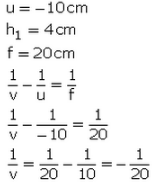v = - 20 cm (Image is 20 cm infront of the convexlens)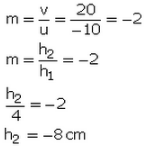Image is 8 cm in size and is real and inverted.

Question 10: A small object is so placed in front of a convex lens of 5 cm focal length that a virtual image is formed at a distance of 25 cm. Find the magnification.

Solution :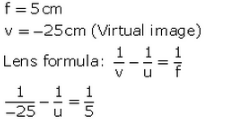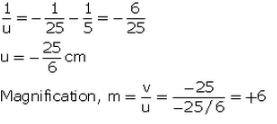Question 11: Find the position and nature of the image of an object 5 cm high and 10 cm in front of a convex lens of focal length 6 cm.

Solution :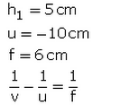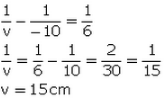Image is formed 15cm behind the convex lens and it is real and inverted.

Question 12: Calculate the focal length of a convex lens which produces a virtual image at a distance of 50 cm of an object placed 20 cm in front of it.

Solution :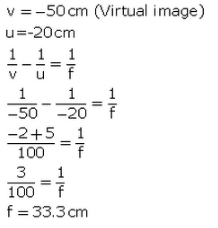Question 13: An object is placed at a distance of 100 cm from a converging lens of focal length 40 cm.
(i) What is the nature of image ?
(ii) What is the position of image ?
A convex lens produces an inverted image magnified three times of an object placed at a distance of 15 cm from it. Calculate focal length of the lens.

Solution :

(i) Since the object Is placed at a distance greater than the focal length of the lens, so the Image formed Is real and inverted.

(ii) u = - 100cm. f = 40cm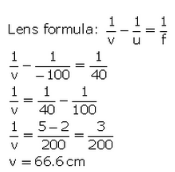Image is formed 66.6cm behind the convex lens.

Question 14: A convex lens produces an inverted image magnified three times of an object placed at a distance of 15 cm from it. Calculate focal length of the lens.

Solution :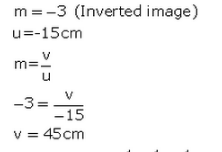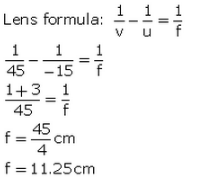Page No:247

Question 15: A converging lens of focal length 5 cm is placed at a distance of 20 cm from a screen. How far from the lens should an object be placed so as to form its real image on the screen ?

Solution :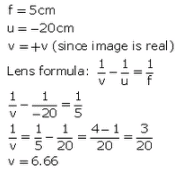Question 16: An object 5 cm high is held 25 cm away from a converging lens of focal length 10 cm. Find the position, size and nature of the image formed. Also draw the ray diagram.

Solution :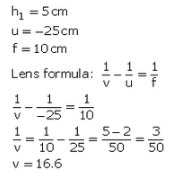Image is 16.6cm behind the oonvex lens.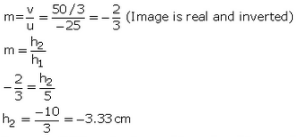Image is 3.33 cm in size and Is real and inverted.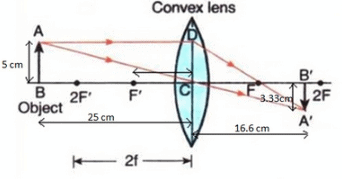Question 17: At what distance should an object be placed from a convex lens of focal length 18 cm to obtain an image at 24 cm from it on the other side ? What will be the magnification produced in this case ?

Solution :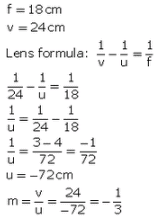Question 18: An object 2 cm tall is placed on the axis of a convex lens of focal length 5 cm at a distance of 10 m from the optical centre of the lens. Find the nature, position and size of the image formed. Which case of image formation by convex lenses is illustrated by this example ?

Solution :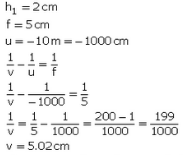The image is formed 5.02cmbehind die convex lens and is real and inverted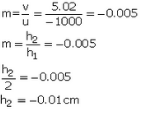Since the object distance is much greater than the focal length, this example illustrates the case when the object is place

Question 19: The filament of a lamp is 80 cm from a screen and a converging lens forms an image of it on a screen, magnified three times. Find the distance of the lens from the filament and the focal length of the lens.

Solution : -u + v = 80cm------(1)
m = -3 (The image is real, since it firms en a screen)
m = v/u = -3
v = -3u

Put in eq (1),
u = -3u = 80
-4u = 80
u = -20cm
Distance of lens from filament is 20cm.
v = -3u = 60cm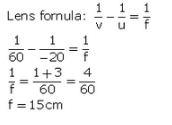Question 20: An erect image 2.0 cm high is formed 12 cm from a lens, the object being 0.5 cm high. Find the focal length of the lens.

Solution :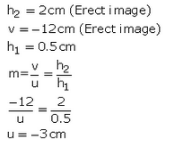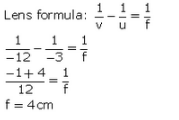Question 21: A convex lens of focal length 0.10 m is used to form a magnified image of an object of height 5 mm placed at a distance of 0.08 m from the lens. Calculate the position, nature and size of the image.

Solution :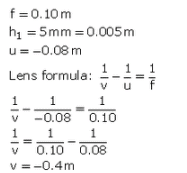Image is formed 0.40 m in front of the convex lens.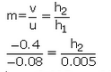h2 = 0.025m = 25mm
Size of image is 25 mm.
Image is virtual and erect

Question 22: A convex lens of focal length 6 cm is held 4 cm from a newspaper which has print 0.5 cm high. By calculation, determine the size and nature of the image produced.

Solution :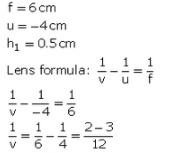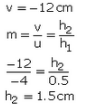Image is 1.5 cm high, virtual, erect and magnified.

Question 23: Determine how far an object must be placed in front of a converging lens of focal length 10 cm in order to produce an erect (upright) image of linear magnification 4.

Solution :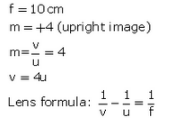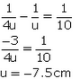The object must be placed 7.5 cm in front of the converging lens.

Question 24: A lens of focal length 20 cm is used to produce a ten times magnified image of a film slide on a screen. How far must the slide be placed from the lens ?

Solution :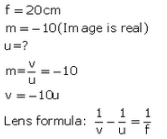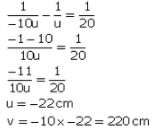Question 25: An object placed 4 cm in front of a converging lens produces a real image 12 cm from the lens.
(a) What is the magnification of the image ?
(b) What is the focal length of the lens ?
(c) Draw a ray diagram to show the formation of image. Mark clearly F and 2F in the diagram.

Solution :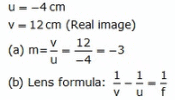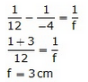Question 26: (a) An object 2 cm tall stands on the principal axis of a converging lens of focal length 8 cm. Find the position, nature and size of the image formed if the object is :
(i) 12 cm from the lens
(ii) 6 cm from the lens
(b) State one practical application each of the use of such a lens with the object in position (i) and (ii).

Solution :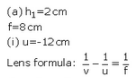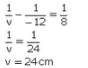Image is 24 cm behind the lens.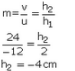Image is 4 an high, real and inverted,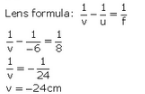Image is 24 cm in front of the lens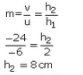Image is 8 cm high, virtual and erect.
(b) (i) Used in film projectcr.
(ii) Used as a magnifying glass.

Question 27: (a) An object 3 cm high is placed 24 cm away from a convex lens of focal length 8 cm. Find by calculations, the position, height and nature of the image.
(b) If the object is moved to a point only 3 cm away from the lens, what is the new position, height and nature of the image ?
(c) Which of the above two cases illustrates the working of a magnifying glass ?

Solution :Image is formed 12 cm behind the lens.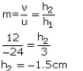Image is l.Scm high, rea! and inverted image is famed 4.8 an In front of the lens.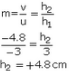Image is 4.8cm high, virtual and erect

(c) Case (b)

Question 28: (a) Find the nature, position and magnification of the images formed by a convex lens of focal length 0.20 m if the object is placed at a distance of :
(i) 0.50 m
(ii) 0.25 m
(iii) 0.15 m
(b) Which of the above cases represents the use of convex lens in a film projector, in a camera, and as a magnifying glass ?

Solution :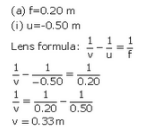Image is formed 0.33 m behind the lens image is formed 1 m behind tfie lens image is formed 0.60 m in front of die lens.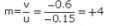Image is virtual and erect
(b) Film projector Case (ii)
Camera: Case (i)
Magnifying glass: Case (iii)

Page No:248

Question 41: A student did an experiment with a convex lens. He put an object at different distances 25 cm, 30 cm, 40 cm, 60 cm and 120 cm from the lens. In each case he measured the distance of the image from the lens. His results were 100 cm, 24 cm, 60 cm, 30 cm and 40 cm, respectively. Unfortunately his results are written in wrong order.
(a) Rewrite the image distances in the correct order.
(b) What would be the image distance if the object distance was 90 cm ?
(c) Which of the object distances gives the biggest image ?
(d) What is the focal length of this lens ?

Solution : (a) 100 cm; 60 cm; 40 cm; 30 cm; 24 cm
(b) When u = -25cm, v = 100cm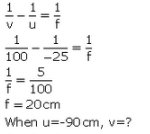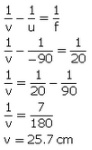(c) 25 cm
(d) 20 cm (As calculated in part (b))

Question 42: A magnifying lens has a focal length of 100 mm. An object whose size is 16 mm is placed at some distance from the lens so that an image is formed at a distance of 25 cm in front of the lens.
(a) What is the distance between the object and the lens ?
(b) Where should the object be placed if the image is to form at infinity ?

Solution :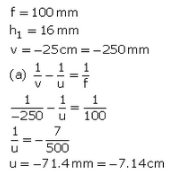Distance between object and lens Is 7.14 cm.

(b)The object shculd be placed at the focus so that the image is formed at infinity.

So, u = -100 mm = -10cm

The object should be placed 10 cm In front of the lens.

Question 43: A lens forms a real image 3 cm high of an object 1 cm high. If the separation of object and image is 15 cm, find the focal length of the lens.

Solution :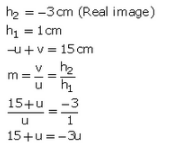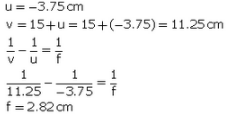Page No:249

Question 44: An object 50 cm tall is placed on the principal axis of a convex lens. Its 20 cm tall image is formed on the screen placed at a distance of 10 cm from the lens. Calculate the focal length of the lens.

Solution :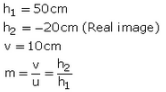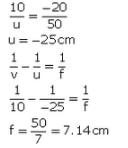Page No:251

Question 1: If the image formed by a lens is always diminished and erect, what is the nature of the lens ?

Solution : Concave lens.

Question 2: Copy and complete the diagram below to show what happens to the rays of light when they pass through the concave lens :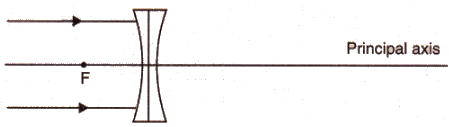Solution :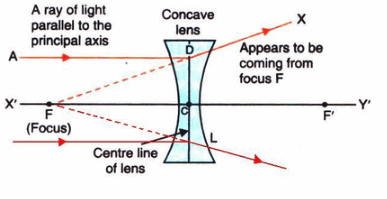Page No:252

Question 3: Which type of lenses are :
(a) thinner in the middle than at the edges ?
(b) thicker in the middle than at the edges ?

Solution : (a) Concave lenses.
(b) Convex lenses.

Question 4: A ray of light is going towards the focus of a concave lens. Draw a ray diagram to show the path of this ray of light after refraction through the lens.

Solution :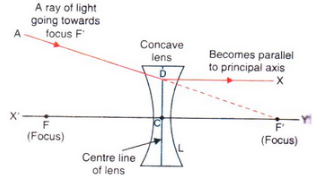Ray of light going towards the focus of a concave lens.

Question 5: (a) What type of images can a convex lens make ?
(b) What type of image is always made by a concave lens ?

Solution : (a) Real and virtual.
(b) Virtual.

Question 6: Take down this figure into your answer book and complete the path of the ray.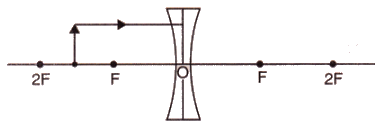Solution :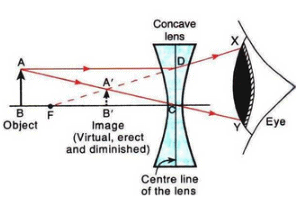Question 7: Fill in the following blanks with suitable words :
(a) A convex lens……. rays of light, whereas a concave lens……….. rays of light.
(b) Lenses refract light to form images : a……… lens can form both real and virtual images, but a diverging
lens forms only……….. images.

Solution : (a) converges; diverges
(b) converging; virtual

Question 8: Things always look small on viewing through a lens. What is the nature of the lens ?

Solution : Concave lens.

Question 9: An object lies at a distance of 2ƒ from a concave lens of focal length ƒ. Draw a ray-diagram to illustrate the image formation.

Solution :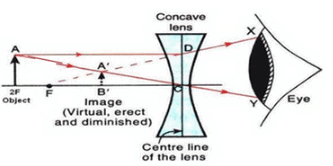Question 10: Show by drawing a ray-diagram that the image of an object formed by a concave lens is virtual, erect and diminished.

Solution :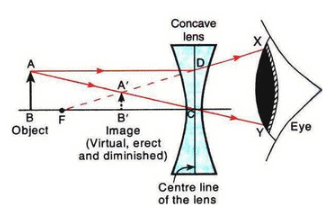Question 11: Give the position, size and nature of image formed by a concave lens when the object is placed :
(a) anywhere between optical centre and infinity.
(b) at infinity.

Solution : (a) When the object is placed anywhere between optical centre and infinity, the image is formed between optical centre and focus. It is diminished, virtual and erect.
(b) When the object is placed at infinity, the image is formed at focus. It is highly diminished, virtual and erect.

Question 12: Which type of lens is : (a) a converging lens, and which is (b) a diverging lens ? Explain your answer with diagrams.

Solution : (a) A convex lens is a converging lens because it converges a parallel beam of light rays passing through it at its focus.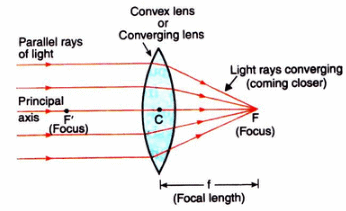Figure — A convex lens converges (brings closer) a parallel beam of light rays to a point F on its other side (right side).

(b) A concave lens is a diverging lens because it diverges the parallel beam of rays passing through it.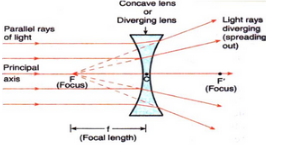Figure — A concave lens diverge  (spreads out) a parallel beam of light rays.

Question 13: With the help of a diagram, explain why the image of an object viewed through a concave lens appears smaller and closer than the object.

Solution : (a) Smaller.
(b) Bigger.
Image is virtual in both the cases.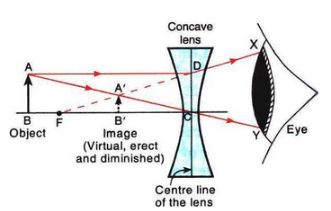As shown by the diagram, the image of an object viewed through a concave lens appears smaller and closer than the object.

Question 14: How would a pencil look like if you saw it through (a) a concave lens, and (b) a convex lens ? (Assume the pencil is close to the lens). Is the image real or virtual ?

Solution :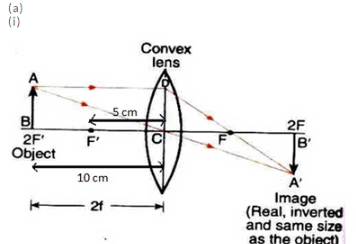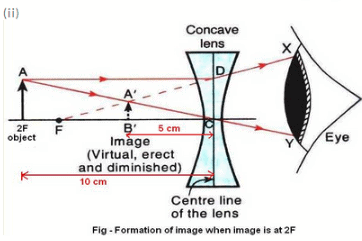(b) Use of convex mirror: As rear-view mirror in vehicles
Use of concave mirror: As shaving mirrors
Use of convex lens: For making simple camera
Use of concave lens: As eye-lens in Galilean telescope

Question 15: (a) An object is placed 10 cm from a lens of focal length 5 cm. Draw the ray diagrams to show the formation
of image if the lens is (i) converging, and (ii) diverging.
(b) State one practical use each of convex mirror, concave mirror, convex lens and concave lens.

Solution :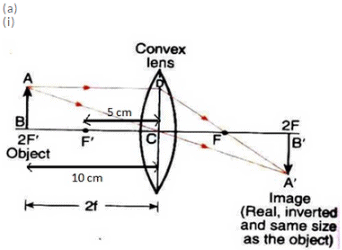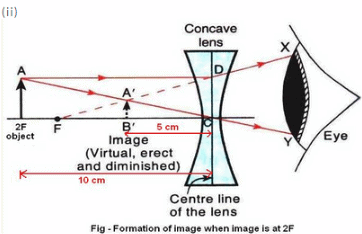(b) Use of convex mirror: As rear view mirror in vehicles
Use of concave mirror: As shaving mirrors
Use of convex lens: For making simple camera
Use of concave lens: As eye-lens in Galilean telescope

Question 16: (a) Construct ray diagrams to illustrate the formation of a virtual image using (i) a converging lens, and
(ii) a diverging lens.
(b) What is the difference between the two images formed above ?

Solution :(a)

(i) Formation of virtual image using a converging lens: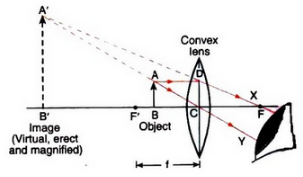(ii) Formation of virtual image using a diverging lens;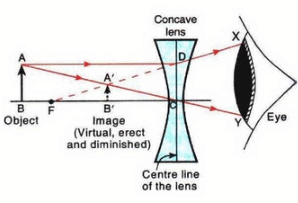(b) The virtual image formed by a converging lens is magnified whereas that formed by a diverging lens is diminished.

Offer running on EduRev: Apply code STAYHOME200 to get INR 200 off on our premium plan EduRev Infinity!

61 docs

,

,

,

,

,

,

,

,

,

,

,

,

,

,

,

,

,

,

,

,

,

,

,

,

;